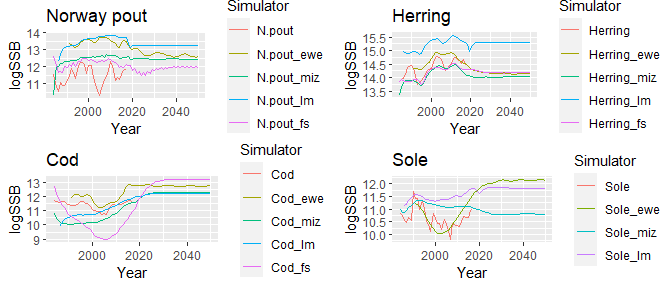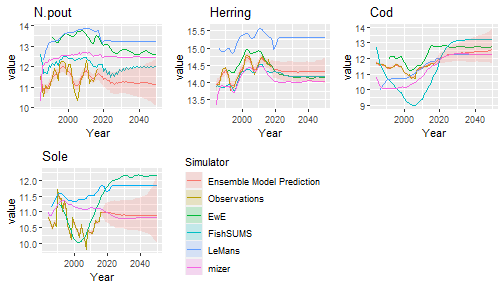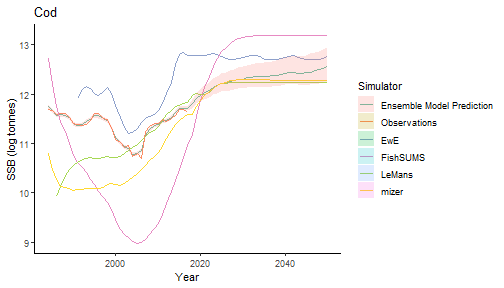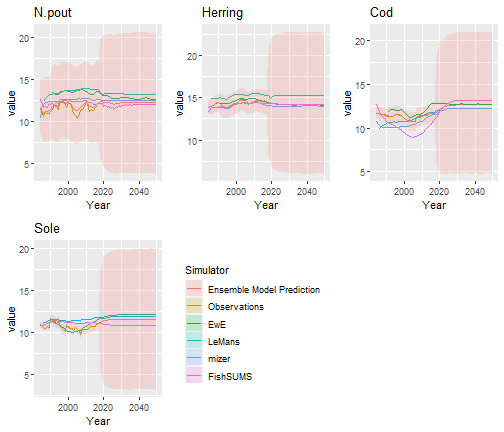# Introduction

When managing ecosystems, management decisions are often informed by outputs of complex ecosystem models (henceforth referred to as simulators). There are often several such simulators available, each providing different outputs with potentially different implications for management actions. While some simulators are better at capturing some aspects of the ecosystem than others, in general no simulator is uniformly better than the others (Chandler (2013)), and often the average of simulators outperforms all of the simulators individually (Rougier (2016)).

Management decisions on the basis of a single simulator are therefore very sensitive to the choice of simulator ( Collie et al. (2016), Essington and Plaganyi (2013), Fulton, Smith, and Johnson (2003), Hart and Fay (2020), Gaichas (2008)) and by ignoring other available simulators, we limit the amount of information utilised and increase our uncertainty (Spence et al. (2018)), often to a greater extent than we formally recognise.

Instead of choosing one simulator, it is possible to combine them using an ensemble model. However, many such methods, such as weighting schemes (e.g. Bayesian model averaging, Banner and Higgs (2017), Dormann, et al. (2018)), explicitly assume that one of the simulators correctly describes the truth (Chandler (2013)). Not only is this a strong assumption, but treating a suite of simulators as containing the true model tends to underestimate the uncertainty because alternative, possibly as yet undeveloped, simulators could give predictions outside of the current range (Chandler (2013)). Under this scheme, adding a new simulator to the suite could increase the uncertainty of the ensemble despite the additional information contained in the new model (Dormann, et al. (2018)). For an ensemble model with a robust quantification of uncertainty, uncertainty should decrease as simulators are added (or at worst stay the same).

Spence et al. (2018) developed an ensemble model treating individual simulators as exchangeable and drawn from a population of possible simulators. They separated each individual simulator’s discrepancy into a discrepancy shared between the simulators and discrepancy that is specific to the simulator. This scheme allows simulator discrepancies that are not independent, which is expected since individual simulator are often similar, having similar processes, forcing inputs or fitted using similar data (Rougier and Beven (2013)). By modelling in this way, the ensemble model exploits the strengths whilst discounting the weaknesses of each individual simulator (Chandler (2013)), whilst robustly quantifying the uncertainty allowing for more accurate advice.

EcoEnsemble is an R package that implements the ensemble model of Spence et al. (2018) when the simulators either output the quantity of interest or not.

# Model description

At time $$t$$, the true quantity of interest for $$d$$ variables, $$\mathbf{y}^{(t)}=(y_1^{(t)},\ldots,y_d^{(t)})'$$, is described by $$m$$ simulators, $$\mathbf{\hat{x}}_{k}^{(t)}=(\hat{x}_{k,1}^{(t)},\ldots,\hat{x}_{k,n_k}^{(t)})'$$, with $$n_k$$ outputs each relating to one of the variables of interest, for $$k=1,\ldots,m$$, and noisy observations of the variables of interest, $$\hat{\mathbf{y}}^{(t)}=(\hat{y}_1^{(t)},\ldots,\hat{y}_d^{(t)})'$$.

Not all of the simulators output all the variables over the whole time period. To accommodate these differences, Spence et al. (2018) introduced a latent variable, known as the “best guess”, $$\mathbf{x}_{k}^{(t)}=({x}_{1}^{(t)},\ldots,{x}_{d}^{(t)})'$$, which represents simulator $$k$$’s output if it described all $$d$$ variables at time $$t$$ with no parameter uncertainty. In EcoEnsemble, variables of interest are either present or absent in each simulator, therefore if the $$k$$th simulator was evaluated at time $$t$$, its output is $\mathbf{\hat{x}}_{k}^{(t)}\sim{}N(M_k^{(t)}\mathbf{x}_{k}^{(t)},\Sigma_k),$ where $$M_k^{(t)}$$ is an $$n_k\times{}d$$ matrix and $$\Sigma_k$$ reflects the parameter uncertainty of the $$k$$th simulator.

The true values of the variables of interest at time $$t$$ is simulator $$k$$’s “best guess” plus a discrepancy term, $$\mathbf{\zeta}^{(t)}_k$$, Kennedy and O’Hagan (2001), i.e.  $\mathbf{y}^{(t)} = \mathbf{x}_{k}^{(t)} + \mathbf{\zeta}^{(t)}_k.$ The discrepancy term, $$\mathbf{\zeta}^{(t)}_k$$, is split between discrepancies that are shared between all of the simulators, and discrepancies that were specific to the $$k$$th simulator. These two discrepancies are further split into fixed discrepancies, the long-term shared discrepancy, $$\mathbf\delta$$, and simulator $$k$$’s long-term individual discrepancy, $$\mathbf\gamma_k$$, and dynamic discrepancies, the short-term shared discrepancy, $$\mathbf\eta^{(t)}$$, and simulator $$k$$’s short-term individual discrepancy, $$\mathbf{z}_k^{(t)}$$, i.e. $\mathbf{\zeta}^{(t)}_k = \mathbf\delta + \mathbf\eta^{(t)} + \mathbf\gamma_k + \mathbf{z}_k^{(t)}.$ The long-term individual discrepancy for the $$k$$th simulator is $\mathbf{\gamma}_k\sim{}N(\mathbf{0},C_{\gamma}).$ The short-term discrepancy terms, $$\mathbf\eta^{(t)}$$ and $$\mathbf{z}_k^{(t)}$$, follow an auto-regressive processes of order one, $\mathbf{\eta}^{(t)}\sim{}N(R_{\eta}\mathbf{\eta}^{(t-1)},\Lambda_{\eta})$ and $\mathbf{z}_{k}^{(t)}\sim{}N(R_{k}\mathbf{z}_{k}^{(t-1)},\Lambda_{k})$ respectively, where the diagonal elements of $$R_i, (i = \eta, 1, 2, \ldots, m )$$ are between $$-1$$ and $$1$$, and the off-diagonal elements are $$0$$.

In the absence of any other information, the true variables evolve according to a random walk, $\mathbf{y}^{(t)}\sim{}N(\mathbf{y}^{(t-1)},\Lambda_y),$ with noisy observations, $\mathbf{\hat{y}}^{(t)}\sim{}N(\mathbf{y}^{(t)},\Sigma_y),$ when there are observations at time $$t$$.

## Dynamic linear model

The ensemble model can be written as a dynamical linear model, by letting $\begin{equation} \mathbf\theta^{(t)}=(\mathbf{y}^{(t)'},\mathbf\eta^{(t)'},\mathbf{z}_1^{(t)'},\ldots,\mathbf{z}_m^{(t)'})', \end{equation}$ so that $\begin{equation} \mathbf\theta^{(t)}|\mathbf\theta^{(t-1)}\sim{}N(A\mathbf\theta^{(t)},\Lambda), \end{equation}$ with $\begin{equation} A= \begin{pmatrix} I_d & 0 & 0 & 0 & \ldots & 0\\ 0 & R_\eta & 0 & 0 & \ldots & 0\\ 0 & 0 & R_1 & 0 & \ldots & 0\\ 0 & 0 & 0 & R_2 & \ldots & 0\\ \vdots & \vdots & \vdots & \vdots & \ddots & \vdots \\ 0 & 0 & 0 & 0 & \ldots & R_m \end{pmatrix}, \end{equation}$ with $$I_d$$ being a $$d$$ dimensional indicator matrix, and $\begin{equation} \Lambda= \begin{pmatrix} \Lambda_y & 0 & 0 & 0 & \ldots & 0\\ 0 & \Lambda_\eta & 0 & 0 & \ldots & 0\\ 0 & 0 & \Lambda_1 & 0 & \ldots & 0\\ 0 & 0 & 0 & \Lambda_2 & \ldots & 0\\ \vdots & \vdots & \vdots & \vdots & \ddots & \vdots \\ 0 & 0 & 0 & 0 & \ldots & \Lambda_m \end{pmatrix}. \end{equation}$ The observations $\begin{equation} \mathbf{w}^{(t)}=(\mathbf{\hat{y}}^{(t)'},\mathbf{\hat{x}}^{(t)'}_1,\ldots,\mathbf{\hat{x}}^{(t)'}_m)' \end{equation}$ are $\begin{equation} S^{(t)}\mathbf{w}^{(t)}\sim{}N(S^{(t)}B(\mathbf{\theta} + \mathbf\zeta),S^{(t)}\Sigma^{(t)}S^{(t)'}) \label{eq:DLM_obs} \end{equation}$ where $\begin{equation} B= \begin{pmatrix} I_d & 0 & 0 & 0 & \ldots & 0\\ M_1 & M_1 & M_1 & 0 & \ldots & 0\\ M_2 & M_2 & 0 & M_2 & \ldots & 0\\ \vdots & \vdots & \vdots & \vdots & \ddots & \vdots \\ M_m & M_m & 0 & 0 & \ldots & M_m \end{pmatrix}, \end{equation}$ $\begin{equation} \mathbf\zeta=(\mathbf{0}',\mathbf{\delta}',\mathbf\gamma'_1,\ldots,\mathbf\gamma'_m)', \end{equation}$ with $$\mathbf{0}$$ being a $$d$$ dimensional vector of 0s, $\begin{equation} \Sigma= \begin{pmatrix} \Sigma_y & 0 & \ldots & 0\\ 0 & \Sigma_1 & \ldots & 0\\ \vdots & \vdots & \ddots & \vdots \\ 0 & 0 & \ldots & \Sigma_m \end{pmatrix} \end{equation}$ and $$S^{(t)}$$ is a matrix that is used to describe which variables are observed at time $$t$$. The $$S^{(t)}$$ matrix is an $$r(t) \times \left(d + \sum_{k=1}^m n_k\right)$$ matrix, where $$r(t)$$ is the total number of observations and simulator outputs available at time $$t$$, with each element given by $S^{(t)}_{i, j} = \begin{cases} 1 & \mathrm{if\ the \ }i^{th} \mathrm{\ available\ observation\ /\ model\ output\ is\ the\ }j^{th}\mathrm{\ of\ all\ possible\ observation\ /\ model\ outputs.} \\ 0 & \mathrm{otherwise} \end{cases}$

For example, if all observations and the simulators give an output at time $$t$$ then $$S^{(t)}=I_{d+\sum_{k=1}^{m}n_k}$$. If all simulators output the variables of interest but there are no observations then $$S^{(t)}$$ is a $$\left(\sum_{k=1}^m n_k\right) \times \left(d + \sum_{k=1}^m n_k\right)$$ matrix, equal to $$I_{d+\sum_{k=1}^{m}n_k}$$ with the first $$d$$ rows removed.

# Dataset for the North Sea

We demonstrate the ensemble model by investigating what would happen to the spawning stock biomass (SSB) of four species in the North Sea ($$d=4$$), Norway pout (Trisopterus esmarkii), Atlantic herring (Clupea harengus), Atlantic cod (Gadus morhua), and Common sole (Solea solea). We used four simulators and estimates of SSB from single-species assessments to predict the true SSB from 1984 to 2017, and the SSB from 2018 to 2050 under MSY.

## Observations

Noisy observations of the SSB were taken from single-species stock assessments (ICES (2020b), ICES (2020a)). The uncertainty is species specific so the off diagonal elements of $$\Sigma_y$$ are zero and the diagonal elements are calculated from the uncertainty in the stock assessments. These values, and the associated uncertainty are contained in the SSB_obs and Sigma_obs variables. The SSB_obs data frame contains a row for each year, with a column for each species observed. Note that for EcoEnsemble, the rows and columns of all observations and model outputs must be named appropriately in order for the data transformations to behave correctly.

SSB_obs
##        N.pout  Herring      Cod     Sole
## 1984 11.53972 13.86278 11.68698 10.82104
## 1985 10.87273 13.94398 11.67321 10.75943
## 1986 10.53821 13.95565 11.59347 10.56377
## 1987 11.07868 14.12404 11.61039 10.46929
## 1988 10.92890 14.38788 11.60985 10.66864
## 1989 10.93916 14.40130 11.52822 10.54621
## 1990 11.19557 14.45303 11.40660 11.70158
## 1991 11.45647 14.32903 11.39265 11.41377
## 1992 11.92440 14.08046 11.35452 11.41658
## 1993 11.70154 13.75959 11.38934 11.00324
## 1994 11.34564 13.82125 11.46735 11.35678
## 1995 12.07449 13.88803 11.61586 11.04353
## 1996 12.24150 13.99439 11.61139 10.55631
## 1997 12.23397 14.11788 11.51042 10.37926
## 1998 12.14652 14.27156 11.48011 10.10641
## 1999 11.59913 14.29834 11.31878 10.82919
## 2000 12.09568 14.33469 11.07164 10.63916
## 2001 12.10364 14.57959 11.02282 10.43383
## 2002 11.38740 14.74728 10.92518 10.43432
## 2003 10.97972 14.77429 10.94854 10.19813
## 2004 10.64428 14.74631 10.72920 10.58993
## 2005 10.33339 14.66267 10.78703 10.38302
## 2006 10.88878 14.45728 10.70340 10.14277
## 2007 11.27246 14.24745 11.24691  9.79971
## 2008 11.45204 14.29349 11.33443 10.44982
## 2009 11.80148 14.46043 11.40682 10.34941
## 2010 12.29722 14.52045 11.39446 10.33850
## 2011 12.07977 14.69997 11.47416 10.30843
## 2012 11.18241 14.75281 11.45062 10.53285
## 2013 11.36872 14.66026 11.50785 10.71819
## 2014 11.27795 14.62658 11.56849 10.65632
## 2015 11.58259 14.53820 11.69435 10.68983
## 2016 11.80500 14.67299 11.69274 10.96791
## 2017 11.93270 14.45042 11.63958 10.98351
Sigma_obs
##             N.pout    Herring         Cod        Sole
## N.pout  0.05754841 0.00000000 0.000000000 0.000000000
## Herring 0.00000000 0.00717835 0.000000000 0.000000000
## Cod     0.00000000 0.00000000 0.005876582 0.000000000
## Sole    0.00000000 0.00000000 0.000000000 0.003132204

## Simulators

EcoPath with EcoSim (EwE) is an ecosystem model with 60 functional groups for the North Sea (ICES (2016)). It outputs all four species from 1991 until 2050, in the variable SSB_ewe, meaning that $$n_1=4$$ and $$M_1=I_4$$. The covariance of the EwE’s output, $$\Sigma_{1}$$, was calculated in Mackinson et al. (2018) and is stored in the variable Sigma_ewe,

Sigma_ewe
##                N.pout      Herring          Cod          Sole
## N.pout   0.0092767506 0.0018710743 8.518305e-04 -1.110854e-04
## Herring  0.0018710743 0.0039672500 4.596743e-04  4.272881e-04
## Cod      0.0008518305 0.0004596743 5.436722e-03  5.581591e-05
## Sole    -0.0001110854 0.0004272881 5.581591e-05  3.140728e-03

LeMans is a discrete time length-based model that describes growth and predation (Thorpe et al. (2015)). It outputs all four species from 1986 until 2050, in the variable SSB_lm, meaning that $$n_2=4$$ and $$M_2=I_4$$. The covariance of the LeMans’ output, $$\Sigma_{2}$$, was calculated in Thorpe et al. (2015) and is stored in the variable Sigma_lm,

Sigma_lm
##              N.pout     Herring         Cod        Sole
## N.pout   0.18223924  0.08142913 -0.02918675  0.12076725
## Herring  0.08142913  0.10463628 -0.01512463  0.13288178
## Cod     -0.02918675 -0.01512463  0.08047544 -0.02147398
## Sole     0.12076725  0.13288178 -0.02147398  0.22548435

mizer is a size-based model that describes ontogenetic feeding and growth, mortality, and reproduction driven by size-dependent predation and maturation processes (Blanchard et al. (2014)). It outputs all four species from 1984 until 2050, in the variable SSB_miz, meaning that $$n_3=4$$ and $$M_3=I_4$$. The covariance of the mizer’s output, $$\Sigma_{3}$$, was calculated in Spence, Blackwell, and Blanchard (2016) and is stored in the variable Sigma_miz,

Sigma_miz
##                N.pout       Herring          Cod          Sole
## N.pout   0.3024728305  0.0324192572 -0.001061507  0.0003276015
## Herring  0.0324192572  0.0680834748  0.023446726 -0.0006754709
## Cod     -0.0010615068  0.0234467261  0.171557394  0.0015845629
## Sole     0.0003276015 -0.0006754709  0.001584563  0.0073544837

FishSUMS is a discrete time length-based model that describes growth, density-dependent mortality, and losses due to fishing and predation by explicitly modelled species, and seasonal reproduction (Speirs, Greenstreet, and Heath (2016)). It outputs Norway pout, Atlantic Herring, and Atlantic Cod (i.e. it does not output Sole), from 1984 until 2050, in the variable SSB_fs, meaning that $$n_4=3$$ and $\begin{equation} M_4= \begin{pmatrix} 1 & 0 & 0 & 0\\ 0 & 1 & 0 & 0\\ 0 & 0 & 1 & 0 \end{pmatrix}. \end{equation}$

The covariance of the FishSUMS’ output, $$\Sigma_{4}$$, was calculated in Spence et al. (2018) and is stored in the variable Sigma_fs. Its value is

Sigma_fs
##               N.pout      Herring           Cod
## N.pout   0.003509705 0.0015517547 -0.0020629595
## Herring  0.001551755 0.0074267464  0.0003581727
## Cod     -0.002062960 0.0003581727  0.0071381322

The single-species stock assessments for the 4 species, along with the model outputs are plotted below.# Fitting the model

## Prior configuration

In EcoEnsemble, short-term discrepancies ($$\mathbf{z_k^{(t)}}, \mathbf{\eta^{(t)}}$$) are modelled using auto-regressive processes of order one, so that \begin{align} \mathbf{z}_{k}^{(t)} & \sim{}N(R_{k}\mathbf{z}_{k}^{(t-1)},\Lambda_{k}), \\ \mathbf{\eta}^{(t)}& \sim{}N(R_{\eta}\mathbf{\eta}^{(t-1)},\Lambda_{\eta}). \end{align} Note also that the long-term individual discrepancies ($$\mathbf{\gamma}_k$$) are drawn from a normal distribution $\begin{equation} \mathbf{\gamma}_k \sim{}N(\mathbf{0},C_{\gamma}). \\ \end{equation}$

To include prior beliefs in the ensemble model, we therefore need to place priors on $$m+2$$ different covariance matrices - $$\Lambda_{1}, \Lambda_2, \ldots \Lambda_m, \Lambda_{\eta}, C_\gamma$$. In EcoEnsemble, the choice of prior parametrisations is the same for all of these matrices, however it should be noted that the interpretation of each is different. In particular, the $$\Lambda$$ matrices are covariances of a dynamic auto-regressive process across time, while $$C_\gamma$$ is the covariance of the distribution of static long-term discrepancies across different simulators.

In EcoEnsemble, we follow the approach of Spence et al. (2018) and decompose the covariance matrices into correlation matrices and a diagonal matrix of variances, i.e.

$\begin{equation} \Lambda = \sqrt{\mathrm{diag}(\mathbf{\pi})} P \sqrt{\mathrm{diag}(\mathbf{\pi})}, \end{equation}$ where $$\mathbf{\pi}$$ is the vector of variances of each species, and $$P$$ is the correlation matrix. Here we write $$\Lambda$$ for any of $$\Lambda_k, \Lambda_\eta, C_\gamma$$.

There are three available prior parametrisations for covariance matrices. In each case, the variance terms are parametrised by inverse-gamma distributions, while the correlation matrices can be parametrised either by an LKJ distribution, an inverse-wishart distribution, or by Beta distributions.

Configuring these options in EcoEnsemble package is most easily done using the EnsemblePrior constructor. A set of default priors is available which produce weakly informative priors that hold for a wide range of different datasets. We can set

#Setting priors for an ensemble model with 4 variables of interest.
priors <- EnsemblePrior(4)

If desired, priors can be manually configured for finer control, for example we can set

priors_custom <- EnsemblePrior(
d = 4,
ind_st_params = IndSTPrior("lkj", list(25, 0.25), 30),
ind_lt_params = IndLTPrior(
"beta",
list(c(25, 25, 25, 10),c(0.25, 0.25, 0.25, 0.1)),
list(matrix(40, 4, 4), matrix(40, 4, 4))
),
sha_st_params = ShaSTPrior("lkj", list(25, 0.25), 30),
sha_lt_params = 3)

This is:

• ind_st_params - The individual short-term discrepancies $$\mathbf{z}_{k}^{(t)}$$. IndSTPrior("lkj", list(25, 0.25), 30) encodes that we use $$\mathrm{\text{Gamma}(25, 0.25)}$$ priors for the variances of all species and an $$LKJ(30)$$ prior on the correlation matrix.
• ind_lt_params - The individual long-term discrepancies $$\mathbf{\gamma_k}$$ use an $$\mathrm{\text{Gamma}(25, 0.25)}$$ prior for the variances of the first 3 species and an $$\mathrm{\text{Gamma}(10, 0.1)}$$ prior for the variance of the final species. The correlation matrix uses a $$\mathrm{Beta}(40, 40)$$ distribution for each of the off-diagonal elements.
• sha_st_params - The shared short-term discrepancies $$\mathbf{\eta}^{(t)}$$ use $$\mathrm{\text{Gamma}(25, 0.25)}$$ priors for variances of all species and an $$LKJ(30)$$ prior on the correlation matrix.
• sha_lt_params - Unlike the other discrepancies, the shared long-term discrepancy $$\mathbf{\mu}$$ is not decomposed into correlation matrices and variances. Instead, a Gaussian distribution is used as the prior on each of the species. sha_lt_params encodes that the standard deviation of this Gaussian prior is 3.

## Model fitting

The ensemble model parameters are fit using the fit_ensemble_model function, which converts the data into the required form. Hamiltonian Monte Carlo then samples from the posterior distribution using the rstan package Stan Development Team (2020). A sample of the ensemble parameters can then be generated using the generate_sample function.

fit_sample <- fit_ensemble_model(observations = list(SSB_obs, Sigma_obs),
simulators = list(list(SSB_ewe, Sigma_ewe, "EwE"),
list(SSB_lm,  Sigma_lm,  "LeMans"),
list(SSB_miz, Sigma_miz, "mizer"),
list(SSB_fs,  Sigma_fs,  "FishSUMS")),
priors = priors)
samples <- generate_sample(fit_sample)

Note that for the simulators, we pass through the name of the simulators as the third argument. This is not required, however provides more accurate error messages and appropriately labeled plots when needed.

## Results

Results can be shown by calling plot on an EnsembleSample object. This plots the observations, simulator outputs, and the ensemble model prediction for the truth for each of the variables of interest. The ensemble model prediction also shows ribbons for the quantiles if a full sampling is performed (by default these are the 5% and 95% quantiles).

plot(samples)We can change which variable we plot by passing through a variable option, and are free to change the aesthetics in the same way as other ggplot2 plots, see the ggplot manual Wickham (2016). Different quantiles can also be specified with the quantiles option, e.g.

plot(samples, variable = "Cod", quantiles = c(0.25, 0.75)) +
ggplot2::theme_classic() +
ggplot2::scale_color_brewer(palette="Set2")  +
ggplot2::ylab("SSB (log tonnes)")# Case Studies

## Visualising priors

Priors can be visualised using the prior_ensemble_model() function and/or the sample_prior() function. These models are quick to evaluate and indicate what restrictions the priors place on the ensemble outputs, for example using the priors from the previous section.

prior_model <- prior_ensemble_model(priors = priors, M = 4)
prior_samples <- sample_prior(observations = list(SSB_obs, Sigma_obs),
simulators = list(list(SSB_ewe, Sigma_ewe, "EwE"),
list(SSB_lm,  Sigma_lm,  "LeMans"),
list(SSB_miz, Sigma_miz, "mizer"),
list(SSB_fs,  Sigma_fs,  "FishSUMS")),
priors = priors, prior_model)

The sample_prior() function produces an EnsembleSample object which can be plotted in the same way as other EnsembleSample objects.

plot(prior_samples)## Finer fitting control

Using the wrapper functions fit_ensemble_model, generate_sample is the easiest way to generate samples from the ensemble model. However, for finer control, the model can be fit directly with rstan. To create data in the correct form of the model, simply create an EnsembleData object using the constructor.

ens_data <- EnsembleData(observations = list(SSB_obs, Sigma_obs),
simulators = list(list(SSB_ewe, Sigma_ewe, "EwE"),
list(SSB_lm,  Sigma_lm,  "LeMans"),
list(SSB_miz, Sigma_miz, "mizer"),
list(SSB_fs,  Sigma_fs,  "FishSUMS")),
priors = priors)

The EnsembleData object has 4 slots: observations, simulators, priors, and stan_input. The first 3 slots are the data as passed through in the constructor. The stan_input slot is automatically generated by transforming the other data into the form required for the Stan implementation of the ensemble model. To fit the model, the compiled rstan object can be retrieved through the get_mcmc_ensemble_model() method.

mod <- get_mcmc_ensemble_model()
samples <- rstan::sampling(mod, data = ens_data@stan_input)
fit <- EnsembleFit(ens_data, samples = samples)

To generate individual samples of the ensemble parameters with this output, now call the get_transformed_data and gen_sample functions:

# Generating samples using DIY functions
transf_data <- get_transformed_data(fit)
mle_sample <- gen_sample(1, ex.fit = rstan::extract(samples), transformed_data = transf_data,
time = ens_data@stan_input\$time)

The equivalent code using wrapper functions:

fit <- fit_ensemble_model(observations = observations, simulators = simulators,
priors = priors)
samples_tmp <- generate_sample(fit)
samples <- samples_tmp@samples